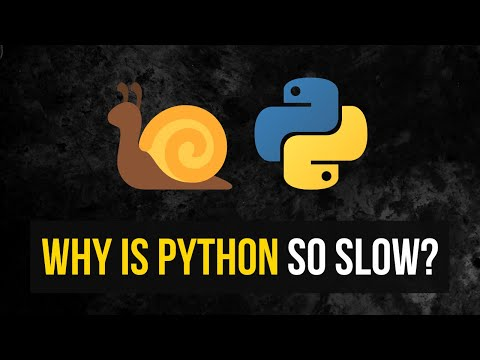# Why is Python So Slow & Does it Matter?In this video we are going to discuss the fundamental question of why Python is so slow and if it actually matters.

In this video we are going to discuss the fundamental question of why Python is so slow and if it actually matters.

Timestamps:
(0:00) Intro
(0:17) Fundamentals
(3:32) Reason 1 - Interpreted
(7:14) Reason 2 - GIL
(11:54) Reason 3 - Dynamic Typing
(14:57) Does it Matter?
(19:20) Outro